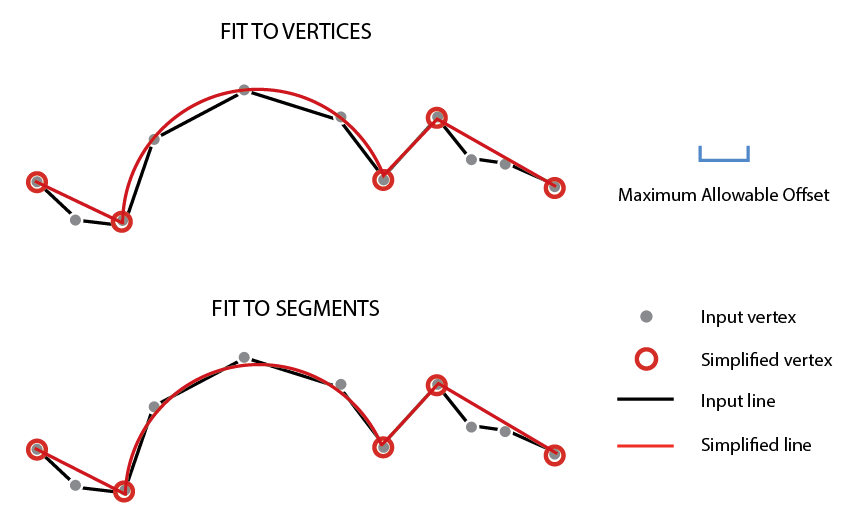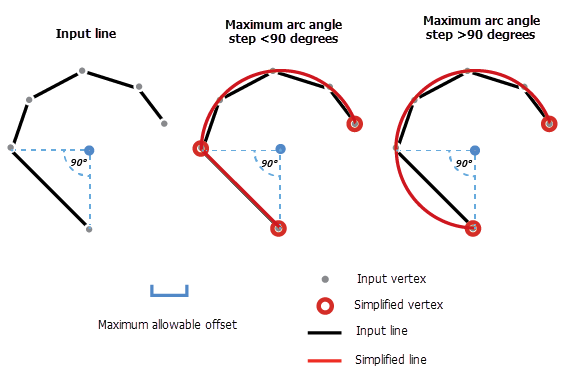# Simplify By Straight Lines And Circular Arcs (Editing)

## Summary

Simplifies polygon and line features by replacing consecutive line segments or edges with fewer line segments or edges. Lines segments and polygon edges are simplified based on a specified maximum allowable offset. Additionally, circular arcs can be created from consecutive line segments or polygon edges.

## Illustration## Usage

##### Caution:

This tool modifies the input data. See Tools that modify or update the input data for more information and strategies to avoid undesired data changes.

• This tool can be used to simplify the geometry of line and polygon features that have accumulated unwanted vertices or short segments from various data conversion and manipulation processes. Sequences of nonlinear segments or edges can be replaced by two-point straight lines, and a series of segments or edges representing arcs can be replaced by true curves. This tool will approximate and replace multiple segments or edges with straight lines and circular arcs that fit within a specified maximum allowable offset.

• Input features can be polygons or lines. The output line or polygon edge contains a subset of its original vertices. New vertices are not introduced and no features are deleted.

• Existing parametric curves, such as Bézier curves, circular arcs, and elliptical arc segments will not be changed.

##### Note:

If the input features are stored in a shapefile, circular arcs in the output features will remain a densely populated series of small straight segments. Shapefiles do not support true curves. To derive true curves, export the shapefile to a feature class.

• Use the Create circular arcs parameter to generate true curves in addition to straight lines. The five optional parameters, Maximum Arc Angle Step, Minimum Number Of Vertices, Minimum Radius, Maximum Radius, and Minimum Arc Angle, control how circular arcs are fitted to segments or edges.

• Maximum Arc Angle Step (decimal degrees) is the maximum arc angle that can be used to construct circular arcs. The arc angle is the central angle of the candidate curve (the curve that is being constructed). The maximum arc angle step defines the upper limit of how wide the central angle search field can be when locating vertices to construct circular curves. If more than one vertex is found within each maximum arc angle step, these vertices will be considered for fitting to a circular arc. Use a smaller arc angle to fit circular arcs to densely distributed segments and to prevent the over-fitting of circular arcs to large segments. Use a larger arc angle to fit circular arcs to sparsely distributed segments. The valid value range is from 2 through 95 decimal degrees. The default is 20 decimal degrees.

In the graphic below, a segment with a 90 degree central angle is considered for the fitting of a circular arc. If 89 degrees is specified as the maximum arc angle step, the segment is not considered and the output consists of a curve and a straight line (middle diagram). If 91 degrees is specified, the segment is used to construct the circular arc, and the output consist of a single curve (end diagram).The Maximum Arc Angle Step parameter is used to locate vertices to construct circular arcs.

• ##### Note:

The Maximum Arc Angle Step parameter is not available if the Fit to segments option is chosen for the Fitting Type parameter.

• Check the Preserve endpoints for closed line parameter to preserve the endpoints of a line that has coincident end points (loop). If Preserve endpoints for closed line is unchecked, the endpoints can be moved or deleted.

## Syntax

`arcpy.edit.SimplifyByStraightLinesAndCircularArcs(in_features, max_offset, {fitting_type}, {circular_arcs}, {max_arc_angle_step}, {min_vertex_count}, {min_radius}, {max_radius}, {min_arc_angle}, {closed_ends}, {anchor_points})`
 Parameter Explanation Data Type in_features[in_features,...] The features to be simplified. Features can be lines or polygons. If using multiple inputs, the features must have the same spatial reference. Feature Layer max_offset The maximum distance the output feature edges can deviate from the input feature shapes. When the Fit to vertices option is chosen for the Fitting Type parameter, the distance is measured between the input vertices and the output feature edges. When the Fit to segments option is chosen, the distance is measured between the input feature edges and the output feature edges. Linear Unit fitting_type(Optional) Specifies how the output feature edges and circular arcs will be fitted to the input feature shapes.If Fit to segments is chosen, the Maximum Arc Angle Step and Minimum Number Of Vertices parameters are not available.FIT_TO_VERTICES —The offset gap between the output feature edges and the input feature vertices will be minimized. Output feature edges and curves will be fitted approximately to the input feature vertex positions. This is the default.FIT_TO_SEGMENTS —The offset gap between the output feature edges and input feature edges will be minimized. Output edges and curves will be fitted approximately to the positions of the input feature shapes. String circular_arcs(Optional) Specifies whether circular arcs will be created.CREATE —Circular arcs will be created. This is the default.NOT_CREATE —Circular arcs will not be created. Boolean max_arc_angle_step(Optional) The maximum arc angle step (decimal degrees) that will be used to construct circular arcs. The arc angle defines how wide the visual field can be, for each step, when locating vertices to construct circular curves. The arc angle is the central angle of the candidate curve (the curve that is being constructed). If vertices are found within each maximum arc angle step, a circular arc is constructed. For example, if vertices and edges are sparse, use a large arc angle step. The valid value range is from 2 through 95 decimal degrees. The default is 20 decimal degrees. This parameter is not available if the Fit to segments option is chosen for the Fitting Type parameter. Double min_vertex_count(Optional) The minimum number of vertices required for a circular arc to be created. The value must be greater than 3. The default is 4. This parameter is not available if the Fit to segments option is chosen for the Fitting Type parameter. Long min_radius(Optional) The smallest allowable radius for output circular arcs. The value must be greater than 0 and smaller than the value specified for Maximum Radius. If no value is specified, the radius of the output circular arcs will not be checked (default). Linear Unit max_radius(Optional) The largest allowable radius for output circular arcs. The value must be greater than the value specified for Minimum Radius. If no value is specified, the radius of the output circular arcs will not checked (default). Linear Unit min_arc_angle(Optional) The minimum arc angle (decimal degrees) that will be used to construct circular arcs. The minimum arc angle is the smallest allowable central angle in the output circular arcs. If the central angle of any output circular arc is less than this value, it will not be created. The valid value range is from 2 through 360 decimal degrees. The default is 2 decimal degrees. Double closed_ends(Optional) Specifies whether the endpoints of a closed line will be preserved. A closed line is a line that has coincident end points (loop).PRESERVE —The endpoints of closed lines will e preserved. This is the default.NOT_PRESERVE —The endpoints of closed lines will not be preserved; they can be moved or deleted. Boolean anchor_points(Optional) The path and name of the feature class that contains anchor points. Anchor points overlay vertices on the input features and indicate that they should not be moved or deleted in the simplify process. Feature Layer

#### Derived Output

 Name Explanation Data Type out_feature_class The updated features. Feature Layer out_feature_layers The updated layer names. Feature Layer

## Code sample

SimplifyByStraightLinesAndCircularArcs example 1 (Python window)

The following Python window script demonstrates how to use the SimplifyByStraightLinesAndCircularArcs function to simplify lines.

``````import arcpy
arcpy.env.workspace = "C:\data\data.gdb"
arcpy.edit.SimplifyByStraightLinesAndCircularArcs_edit("myDensifiedLines",
"1.5 feet")``````
SimplifyByStraightLinesAndCircularArcs example 2 (Python window)

The following Python window script demonstrates how to use the SimplifyByStraightLinesAndCircularArcs function to simplify lines and polygons simultaneously with anchor points.

``````import arcpy
arcpy.env.workspace = "C:\data\data.gdb"
arcpy.edit.SimplifyByStraightLinesAndCircularArcs("myDensifiedPolygons;myDensifiedLines",
"0.4 Meters",
anchor_points="myAnchorPoints")``````

## Licensing information

• Basic: No
• Standard: Yes